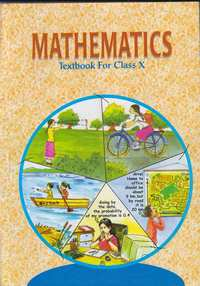Share

# NCERT solutions for Class 10 Mathematics chapter 8 - Introduction to Trigonometry

## Mathematics Textbook for Class 10

#### NCERT Mathematics Class 10## Chapter 8: Introduction to Trigonometry

Ex. 8.30Ex. 8.40

#### Chapter 8: Introduction to Trigonometry Exercise 8.30 solutions [Pages 189 - 190]

Ex. 8.30 | Q 1.1 | Page 189

Evaluate (sin 18^@)/(cos 72^@)

Ex. 8.30 | Q 1.2 | Page 189

Evaluate (tan 26^@)/(cot 64^@)

Ex. 8.30 | Q 1.4 | Page 189

Evaluate cosec 31° − sec 59°

Ex. 8.30 | Q 2.2 | Page 189

Show that cos 38° cos 52° − sin 38° sin 52° = 0

Ex. 8.30 | Q 3 | Page 189

If tan 2A = cot (A – 18°), where 2A is an acute angle, find the value of A

Ex. 8.30 | Q 4 | Page 189

If tan A = cot B, prove that A + B = 90

Ex. 8.30 | Q 5 | Page 189

If sec 4A = cosec (A− 20°), where 4A is an acute angle, find the value of A.

Ex. 8.30 | Q 7 | Page 190

Express sin 67° + cos 75° in terms of trigonometric ratios of angles between 0° and 45°

#### Chapter 8: Introduction to Trigonometry Exercise 8.40 solutions [Pages 193 - 194]

Ex. 8.40 | Q 1 | Page 193

Express the trigonometric ratios sin A, sec A and tan A in terms of cot A.

Ex. 8.40 | Q 2 | Page 193

Write all the other trigonometric ratios of ∠A in terms of sec A.

Ex. 8.40 | Q 3.1 | Page 193

Evaluate

(sin ^2 63^@ + sin^2 27^@)/(cos^2 17^@+cos^2 73^@)

Ex. 8.40 | Q 3.2 | Page 193

Evaluate sin25° cos65° + cos25° sin65°

Ex. 8.40 | Q 4.1 | Page 193

Choose the correct option. Justify your choice.

9 sec2 A − 9 tan2 A =

• 1

• 9

• 8

• 0

Ex. 8.40 | Q 4.2 | Page 193

Choose the correct option. Justify your choice.

(1 + tan θ + sec θ) (1 + cot θ − cosec θ)

• 0

• 1

• 2

• -1

Ex. 8.40 | Q 4.3 | Page 193

Choose the correct option. Justify your choice.

(secA + tanA) (1 − sinA) =

• secA

• sinA

• cosecA

• cosA

Ex. 8.40 | Q 4.4 | Page 193

Choose the correct option. Justify your choice.

(1+tan^2A)/(1+cot^2A)

• secA

• −1

• cotA

• tanA

Ex. 8.40 | Q 5.01 | Page 193

Prove the following identities, where the angles involved are acute angles for which the expressions are defined

(cosec θ – cot θ)^2 = (1-cos theta)/(1 + cos theta)

Ex. 8.40 | Q 5.02 | Page 193

Prove the following identities, where the angles involved are acute angles for which the expressions are defined

cos A/(1 + sin A) + (1 + sin A)/cos A = 2 sec A

Ex. 8.40 | Q 5.03 | Page 194

Prove the following identities, where the angles involved are acute angles for which the expressions are defined

(tantheta)/(1-cottheta) + (cottheta)/(1-tantheta) = 1+secthetacosectheta

Ex. 8.40 | Q 5.04 | Page 194

Prove the following identities, where the angles involved are acute angles for which the expressions are defined.

(1+ secA)/sec A = (sin^2A)/(1-cosA)

[Hint : Simplify LHS and RHS separately]

Ex. 8.40 | Q 5.05 | Page 194

Prove the following identities, where the angles involved are acute angles for which the expressions are defined.

(cos A-sinA+1)/(cosA+sinA-1)=cosecA+cotA

Ex. 8.40 | Q 5.06 | Page 194

Prove the following identities, where the angles involved are acute angles for which the expressions are defined.

sqrt((1+sinA)/(1-sinA)) = secA + tanA

Ex. 8.40 | Q 5.07 | Page 194

Prove the following identities, where the angles involved are acute angles for which the expressions are defined

(sin theta-2sin^3theta)/(2cos^3theta -costheta) = tan theta

## Chapter 8: Introduction to Trigonometry

Ex. 8.30Ex. 8.40

#### NCERT Mathematics Class 10## NCERT solutions for Class 10 Mathematics chapter 8 - Introduction to Trigonometry

NCERT solutions for Class 10 Maths chapter 8 (Introduction to Trigonometry) include all questions with solution and detail explanation. This will clear students doubts about any question and improve application skills while preparing for board exams. The detailed, step-by-step solutions will help you understand the concepts better and clear your confusions, if any. Shaalaa.com has the CBSE Mathematics Textbook for Class 10 solutions in a manner that help students grasp basic concepts better and faster.

Further, we at Shaalaa.com are providing such solutions so that students can prepare for written exams. NCERT textbook solutions can be a core help for self-study and acts as a perfect self-help guidance for students.

Concepts covered in Class 10 Mathematics chapter 8 Introduction to Trigonometry are Trigonometric Ratios of Complementary Angles, Trigonometric Identities.

Using NCERT Class 10 solutions Introduction to Trigonometry exercise by students are an easy way to prepare for the exams, as they involve solutions arranged chapter-wise also page wise. The questions involved in NCERT Solutions are important questions that can be asked in the final exam. Maximum students of CBSE Class 10 prefer NCERT Textbook Solutions to score more in exam.

Get the free view of chapter 8 Introduction to Trigonometry Class 10 extra questions for Maths and can use Shaalaa.com to keep it handy for your exam preparation

S